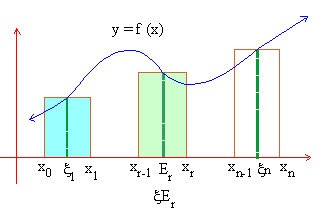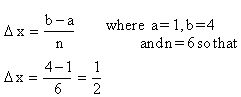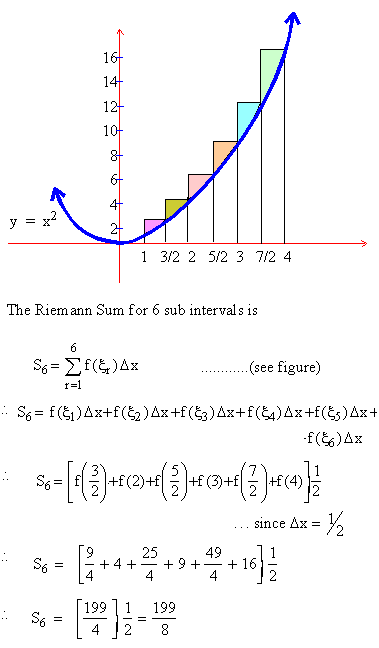Home MonkeyNotes Printable Notes Digital Library Study Guides Study Smart Parents Tips College Planning Test Prep Fun Zone Help / FAQ How to Cite New Title Request

 7.2 Definite Integral as an Area under a Curve Observe the adjoining figure. It is clear that f (xr) Dxr is the area of the rectangle with base Dxr = xr - xr-1 and height f (x r ).If now n ® ¥ , in such a way that the largest of all the sub intervals, Dx1, Dx2, .......Dxn tends to zero, then we should expect that Sn would approximate to the area say A, bounded by the curve y = f (x), the x - axis and the ordinates x = a and x = b. That is lim Sn = A, provided the limit exists .             n ® ¥ Example 1 Evaluate the Riemann Sum for f(x) = x2 on [1, 4] using 6 sub intervals of equal length. Solution : Since the sub intervals are to be of equal lengths, you find thatIndex 7.1 Definition 7.2 Definite integral as on an area under curve 7.3 Working rule for evaluation of a definite integral as a limit of a sun 7.4 Fundamental theorem of integrated calculus 7.5 Properties of definite integrals 7.6 Evaluation of definite integral 7.7 Reduction formulas Chapter 8